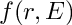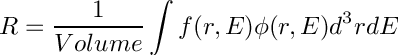MURE
TallyMultiplicator Class Reference

Define a Tally Multiplicator entry (FM card in MCNP, dr card in Serpent). More...

#include <MureTally.hxx>

## Public Member Functions

TallyMultiplicator (Material *M, int reaction, double constant=1)

TallyMultiplicator (Material *M, Reaction *R, double constant=1)

TallyMultiplicator (int MatNumber, int reaction, double constant=1)

TallyMultiplicator (int MatNumber, Reaction *R, double constant=1)
Constructor of Multiplicator Bin. More...

TallyMultiplicator (const TallyMultiplicator &fm)
Copy constructor. More...

~TallyMultiplicator ()
Destructor. More...

int GetMaterialNumber ()

double GetConstant ()

ReactionGetReaction ()

## Private Member Functions

void Init (int MatNumber, Reaction *R, double constant=1)
Build a TallyMultiplicator. Called by constructors. More...

## Private Attributes

double fConstant
multiplicative constant of the FM, not use in Serpent. More...

int fMatNumber
Material Number of the FM/dr card. More...

ReactionfReaction
Reaction of the FM/dr card. More...

## Detailed Description

Define a Tally Multiplicator entry (FM card in MCNP, dr card in Serpent).

A TallyMultiplicator is used to modify the output of a Tally (or detector in Serpent) ; The multiplicator, multiply the tally result by a functionasThe function is generally a reaction, thus the tally response is a reaction rate.

Version
1.0

## ◆ TallyMultiplicator() [1/5]

 TallyMultiplicator::TallyMultiplicator ( Material * M, int reaction, double constant = 1 )

Constructor of Multiplicator Bin. It allows to calculate a reaction rate (flux*sigma) for the given reaction number on the Material M. The constant is not use in Serpent. In MCNP, the reaction rate is multiply by the "constant" parameter but if constant<0 then constant is replaced by |constant|*(atom density of the cell where Tally is wanted). It can be used to calculated macroscopic reaction rates.

Parameters
 M : the Material to consider for the reaction reaction : a simple reaction code (1=total, 18=fission, ...) constant : the multiplicative constant of the FM in MCNP (not use in Serpent, see above). Default=1;

## ◆ TallyMultiplicator() [2/5]

 TallyMultiplicator::TallyMultiplicator ( Material * M, Reaction * R, double constant = 1 )

Constructor of Multiplicator Bin. It allows to calculate a reaction rate (flux*sigma) for the given reaction number on the Material M. The constant is not use in Serpent. In MCNP, the reaction rate is multiply by the "constant" parameter but if constant<0 then constant is replaced by |constant|*(atom density of the cell where Tally is wanted). It can be used to calculated macroscopic reaction rates.

Parameters
 M : the Material to consider for the reaction R : a Reaction constant : the multiplicative constant of the FM (not use in Serpent, see above). Default=1;

## ◆ TallyMultiplicator() [3/5]

 TallyMultiplicator::TallyMultiplicator ( int MatNumber, int reaction, double constant = 1 )

Constructor of Multiplicator Bin. see description of TallyMultiplicator(Material *M, int reaction, double constant=1)

Parameters
 MatNumber : the Material Number to consider for the reaction reaction : a simple reaction code (-1=total, -6=fission, ...) constant : the multiplicative constant of the FM (not use in Serpent, see above). Default=1;

## ◆ TallyMultiplicator() [4/5]

 TallyMultiplicator::TallyMultiplicator ( int MatNumber, Reaction * R, double constant = 1 )

Constructor of Multiplicator Bin.

see description of TallyMultiplicator(Material *M, int reaction, double constant=1)

Parameters
 MatNumber : the Material Number to consider for the reaction R : a Reaction constant : the multiplicative constant of the FM (not use in Serpent, see above). Default=1;

## ◆ TallyMultiplicator() [5/5]

 TallyMultiplicator::TallyMultiplicator ( const TallyMultiplicator & fm )

Copy constructor.

## ◆ ~TallyMultiplicator()

 TallyMultiplicator::~TallyMultiplicator ( )

Destructor.

## ◆ GetConstant()

 double TallyMultiplicator::GetConstant ( )
inline

< a multiplicative constante (not use in Serpent)

## ◆ GetMaterialNumber()

 int TallyMultiplicator::GetMaterialNumber ( )
inline

< return the material used to calculate the reaction

## ◆ GetReaction()

 Reaction* TallyMultiplicator::GetReaction ( )
inline

< The reaction that multiply the tally result.

## ◆ Init()

 void TallyMultiplicator::Init ( int MatNumber, Reaction * R, double constant = 1 )
private

Build a TallyMultiplicator. Called by constructors.

## ◆ fConstant

 double TallyMultiplicator::fConstant
private

multiplicative constant of the FM, not use in Serpent.

## ◆ fMatNumber

 int TallyMultiplicator::fMatNumber
private

Material Number of the FM/dr card.

## ◆ fReaction

 Reaction* TallyMultiplicator::fReaction
private

Reaction of the FM/dr card.

The documentation for this class was generated from the following files:MURE Project, documentation generated by Doxygen 1.9.1 - Mon Jan 3 2022